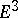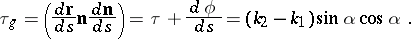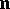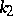# Geodesic torsion

of a curveon a surfaceinThe rate of rotation of the tangent plane toaround the tangent to. The rate is measured with respect to the arc lengthduring the movement of the tangent lines along. The curveand the surfaceare supposed to be regular and oriented. The geodesic torsion onis determined by the points and the direction of the curve and equals the torsion of the geodesic line in that direction. The geodesic torsion is given byHereis the radius vector of the curve;is the unit normal to;is the ordinary torsion of; andis the angle between the osculating plane of the curve and the tangent plane to the surface;andare the principal curvatures of the surface andis the angle between the curve and the direction of.